×

Search anything:

# Space Time Complexity [Computer Science]

#### Algorithms Time ComplexityFREE BOOK -> Problems for the day before your Coding Interview (on Amazon)

In this article at OpenGenus, we have covered the concept of Space Time Complexity in depth which is a must in Algorithmic Analysis.

Table of Content

1. Time Commplexity
2. Big O notation
3. Big Omega
4. Theta Notation
5. little Omega
6. Space complexity
• Auxiliary space
• Space complexity
• Bubble sort time
• selection sort time
• insertion sort time
7. Recursive Algorithms
• Linear
• Divide and Conquer
8. Methods to solve to get complexity

## What is time complexity

It is a mathematical function that tells us relationship about how the time is going to grow as the input grows.

## What do we consider when thinking about complexity

• Always look for worst case complexity
• always look at complexity for large / infinite data
• Even though the value of specific time is different they are all growing linearly.
• we don’t care about actual time. we care about how the relation of time is growing. Because time for different systems will be different.
• This is why we ignore all constants.
• always ignore less dominant terms
E.x.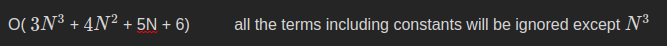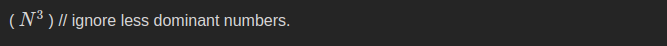### Dominance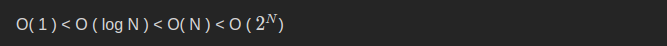## Big O Notation

Big O notation is used in computer science to describe the performance or complexity of an algorithm. It represents the upper bound or worst-case scenario of the algorithm's time or space requirements. It provides a way to compare algorithms and understand their scalability. In simple terms, it shows how the algorithm's efficiency changes as the input size grows.
Also known as Bachmann–Landau notation or asymptotic notation, The letter O was chosen by Bachmann to stand for Ordnung, meaning the order of approximation.

### Word definition

``````O ( N ) → Upper Bound
``````

The time complexity will not exceed the upper limit .

## Maths definition

`````` f(N) = O(g(N))
``````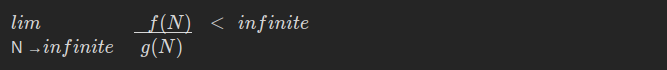For further detail information go to this wikipedia site

# Big Omega Ω

Opposite of big O
Big Omega (Ω) notation is used to describe the lower bound or best-case scenario of an algorithm's time or space complexity. It represents the minimum amount of resources required by the algorithm for a given input size, ensuring that the algorithm will not perform better than this bound.

### Word definition → Opposite of big O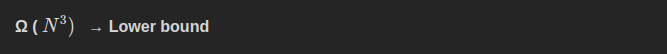Minimum time complexity

## Maths definition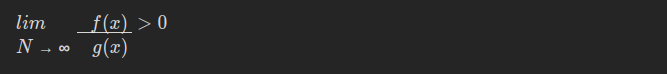### What if an algorithm has lower bound and upper bound as (N^2).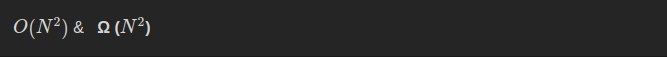## Theta Notation θ

Theta (Θ) notation is used to describe the tight or average-case scenario of an algorithm's time or space complexity. It represents both the upper and lower bounds of the algorithm, indicating that the algorithm's performance will be within a constant factor of the bound for a given input size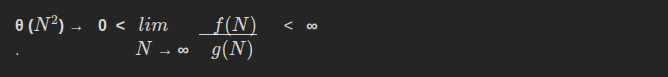In words Both Upper bound & Lower bound = N^2

## Little O Ω

This is also giving loose upper bound

### Maths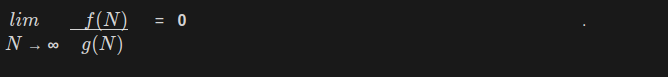``````Big O                             Little O   (stronger statement)

f = O(g)\$                         f = O(g)
f <= g\$                           f < g  strictly slower than g
``````

# Space Complexity or Auxiliary space?

Auxiliary space is the extra space or temporary space used by an algorithms.

Space Complexity of an algorithm is total space taken by the algorithm with respect to the input size. Space complexity includes both Auxiliary space and space used by input.

Bubble Sort time complexity
Bubble Sort is a simple sorting algorithm that repeatedly swaps adjacent elements if they are in the wrong order, gradually pushing the larger elements towards the end of the list.

• worst / average case Time Complexity \$O(N^2).\$
• Best case Time Complexity \$O(n).\$
• Auxiliary Space : O (1)
• Boundary Cases : order of n

Selection Sort Time Complexity
Selection Sort is a sorting algorithm that repeatedly selects the smallest or largest element from the unsorted part of the list and swaps it with the element at the beginning of the sorted part. It continues this process until the entire list is sorted.

• Worst complexity: \$N^2\$
• Average complexity / Best complexity: \$N^2\$
• Space complexity: 1

Insertion Sort Time Complexity
Insertion Sort is an efficient sorting algorithm that builds the final sorted array one element at a time. It iterates through the list, comparing each element to its previous elements and inserting it in the correct position.

Time Complexity: \$O(n^2)
Auxiliary Space: O(1)

Boundary cases: Insertion sort takes maximum time to sort if elements are sorted in reverse order. And it takes minimum time (Order of n), when elements are already sorted.

## Recursive Algorithms

Space complexity in Recursive Algorithms refers to the amount of memory required to execute the algorithm. As an example, consider a recursive algorithm to calculate the factorial of a number. The space complexity would be determined by the maximum depth of the recursive calls, which determines the number of activation records stored on the call stack. The space complexity in this case is O(n), where n is the input size, as each recursive call adds a new activation record to the stack until the base case is reached.

``````def factorial(n):
if n == 0:
return 1
else:
return n * factorial(n-1)
``````

In this code, the factorial() function takes an input n and recursively calculates its factorial. The base case is when n equals 0, in which case the function returns 1. Otherwise, it makes a recursive call to factorial(n-1) and multiplies the result by n. Each recursive call consumes additional memory on the call stack.

### Two types of Recursion

1. Linear
Linear recursion is a recursive algorithmic approach in which a problem is divided into smaller subproblems, and each subproblem is solved before moving on to the next. The solution to the current problem depends on the solution of the previous subproblem, leading to a sequential execution of recursive calls.
`````` f(N) = f(N-1) + f(N-2)
``````
1. Divide and Conquer
Divide and conquer recursion is a recursive algorithmic technique that involves breaking down a problem into smaller, more manageable subproblems, solving them independently, and then combining their solutions to obtain the final result. It divides the problem into non-overlapping subproblems, conquers them by solving independently, and combines their solutions to solve the original problem.
``````f(N) = f{N/2} + O(1)
``````

The divide-and-conquer technique involves taking a large-scale problem and dividing it into similar sub-problems of a smaller scale and recursively solving each of these sub-problems.

Form: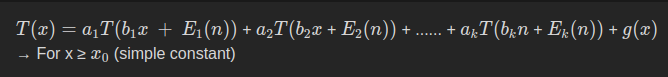### Methods to solve to get complexity

1. Plug and chug
This method involves directly solving the recurrence relation by recursively plugging in values and simplifying until a closed-form expression is obtained. It requires careful analysis and manipulation of the equation to determine the complexity. The constant term (C) represents the non-recursive operations.
``````F(N) = f({N}/{2}) + C
``````
1. Master’s Theorem provides a framework for analyzing recurrence relations of the form T(n) = aT(n/b) + f(n), where a ≥ 1, b > 1, and f(n) is a given function. It provides explicit formulas to determine the time complexity based on the relationship between the function f(n) and the parameters a and b.
2. Akra Bazzi Theorem is a generalization of the Master's Theorem for solving non-homogeneous recurrence relations with variable coefficients. It provides a method to find the time complexity of divide-and-conquer algorithms when the subproblems have different sizes. It involves evaluating an integral expression to determine the overall complexity of the algorithm.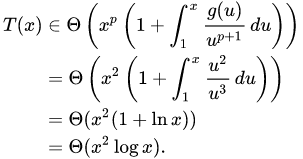#### Manish Kumar

I am first year student at KIIT. I am currently learning Web Development, started writing blogs as to record my learning journey, it gives me deeper understanding when I learn and write it later.

Space Time Complexity [Computer Science]